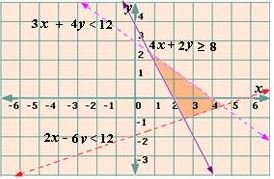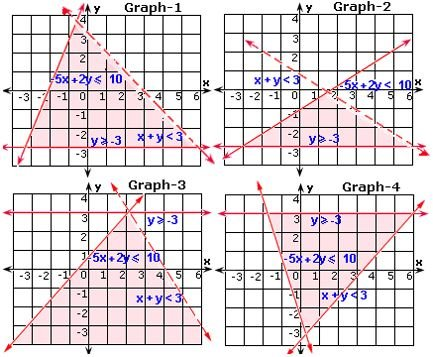## Definition Of System Of Inequalities

A System of Equations is a set of two or more equations with the same variables graphed on the same coordinate plane.

### Examples of System of Inequalities

The figure below shows the graph of the system of inequalities
2x - 6y < 12,="" 3x="" +="" 4y="">< 12,="" and="" 4x="" +="" 2y="" ≥="" 8.="">

### Video Examples: Algebra 2- Solving Systems of Equations### Solved Example on System of Inequalities

#### Ques: Which of the graphs defines the system of inequalities, x + y < 3,="" -="" 5x="" +="" 2y="" ≤="" 10,="" y="" ≥="" -="">##### Choices:

A. Graph-1
B. Graph-2
C. Graph-3
D. Graph-4